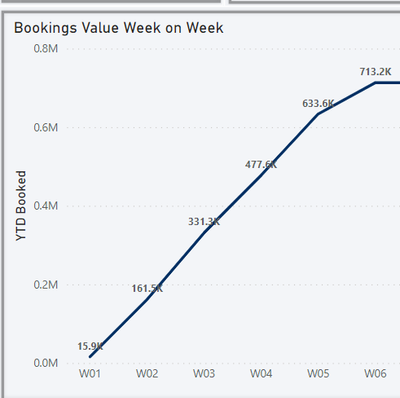cancel
Showing results for
Did you mean:Frequent Visitor

## % increase of Year to Date on the Same Year to date 1 calendar week ago

Good Evening,

Im looking to create a % increase in Week on Week performance, But the weeks must be a running total of the year to date

See below, I would need in W7 to get a % increase from W6 to W7. Below shows a basic

= TOTALYTD(SUM(Booking[Total Price]),CalendarBooked[Date])2 ACCEPTED SOLUTIONSSuper User

Hi,

Assuming Week numbers on the X-axis have been dragged from the Calendar table and there is a relationship (Many to One and Single) from the Date column Booking table to the Date column of the Calendar Table, write these measures:

TP = SUM(Booking[Total Price])

TP YTD = calculate([tp],datesytd(calendar[date],"31/12"))

TP YTD till last week = calculate([tp tyd],datesbetween(calendar[date],min(calendar[date])-7,min(calendar[date])-1))

Growth (%) = divide(([TP YTD]-[TP YTD till last week]),[TP YTD till last week])

Hope this helps.

Regards,
Ashish Mathur
http://www.ashishmathur.comFrequent Visitor

Perfect, thank you. Only thing it doesnt do is exlucde the calc on the first week of the year, so brings in the full year from before, Is there a way to exlclude this?

4 REPLIES 4Super User

Hi,

Assuming Week numbers on the X-axis have been dragged from the Calendar table and there is a relationship (Many to One and Single) from the Date column Booking table to the Date column of the Calendar Table, write these measures:

TP = SUM(Booking[Total Price])

TP YTD = calculate([tp],datesytd(calendar[date],"31/12"))

TP YTD till last week = calculate([tp tyd],datesbetween(calendar[date],min(calendar[date])-7,min(calendar[date])-1))

Growth (%) = divide(([TP YTD]-[TP YTD till last week]),[TP YTD till last week])

Hope this helps.

Regards,
Ashish Mathur
http://www.ashishmathur.comFrequent Visitor

Perfect, thank you. Only thing it doesnt do is exlucde the calc on the first week of the year, so brings in the full year from before, Is there a way to exlclude this?Super User

You are welcome.  Does this measure work?

TP YTD till last week = if(Calendar[Week]="W1",blank(),calculate([tp tyd],datesbetween(calendar[date],min(calendar[date])-7,min(calendar[date])-1)))

Regards,
Ashish Mathur
http://www.ashishmathur.comSuper User

@Johnce1607 , Try like